# Test: Transistor Biasing & Thermal Stabilization- 2

## 15 Questions MCQ Test Topicwise Question Bank for Electrical Engineering | Test: Transistor Biasing & Thermal Stabilization- 2

Description
Attempt Test: Transistor Biasing & Thermal Stabilization- 2 | 15 questions in 45 minutes | Mock test for Electrical Engineering (EE) preparation | Free important questions MCQ to study Topicwise Question Bank for Electrical Engineering for Electrical Engineering (EE) Exam | Download free PDF with solutions
QUESTION: 1

Solution:
QUESTION: 2

Solution:
QUESTION: 3

### For the circuit shown below, what is the value of RC needed if VC = 2 V ?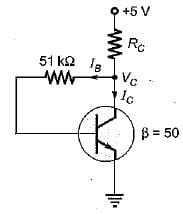Solution:

Using KVL, we have:
-5 + RC (IB + IC) + RBIB + 0.7 = 0
Since, VCE = V= 2 V, therefore transistor is in active mode.
∴ IC = βIB
So,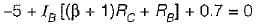or,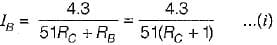Now,.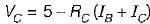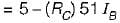or,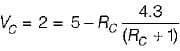(Using equation (i))
or, RC = 2.3 kΩ

QUESTION: 4

Assuming the current source to be ideal, all transistors to be identical, and IC = βIB the stability factor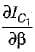for the current mirror circuit shown below would be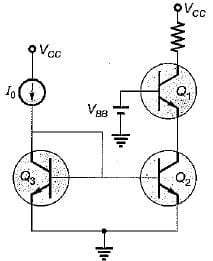Solution:

Applying KCL at the collector of Q3 gives,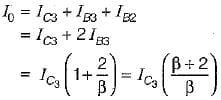or,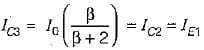Also,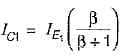Hence,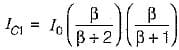Therefore,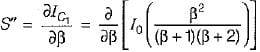or,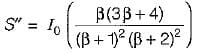QUESTION: 5

A reverse biased diode placed in parallel with the base-emitter junction makes the circuit insensitive to variations in______with changes in temperature.

Solution:

The circuit for the “diode compensation for I"CO is shown below.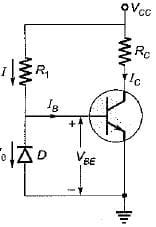Here, if the diode and the transistor are of the same type and material, the reverse saturation current I0 of the diode will increase with temperature at the same rate as the transistor current ICO.
Here,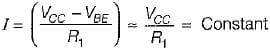Since the diode is reverse biased by VBE = 0.2 V (for Ge device), the current through D is I0 and IB = I - I0.
Now,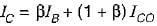Thus, if β ≫ 1 and if I0 of D and ICO of transistor track each other over the desired temperature range, then IC remains essentially constant so that the circuit becomes insensitive toe variations in ICO with changes in temperature.

QUESTION: 6

The static (DC) and dynamic (AC) load lines in a transistor amplifier are different when

Solution:
QUESTION: 7

Thermal runaway is not possible in FET because as the temperature of FET increases,

Solution:
QUESTION: 8

The two transistors in figure are identical. If β = 25, the current IC2 is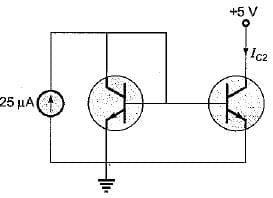Solution:

Both transistor are in the forward active region.
Here, IC1 + IB1 + IB2 = 25 μA
Since the transistors are identical and have the same VBE, therefore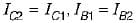∴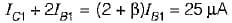or,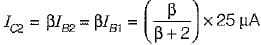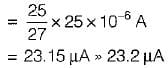QUESTION: 9

The input resistance of a common emitter stage can be increased by
1. unbypassing emitter resistance
2. bootstrapping
3. biasing it at low quiescent current
4. using compounded BJTs

Q. The correct sequence in descending order of the effectiveness of these methods is

Solution:
QUESTION: 10

For the transistor amplifier shown in figure below, VCC = 12 V, R1, = 8 kΩ, R2 = 4 kΩ, RC = 1 kΩ, RE = 1 kΩ.
Assuming VBE = 0.7 V, the operating point Q be given as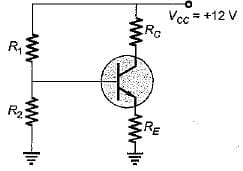Solution:

The voltage across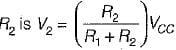Therefore,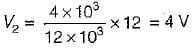Now,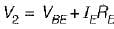∴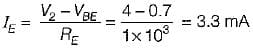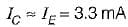∴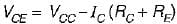= 12 - 3.3 x 10-3 x 2 x 103
= 5.4 volt
∴ Q = (5.4 V, 3.3 mA)

QUESTION: 11

In a self bias circuit using BJT, if the stability factor is represented as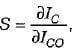then the rate of change of IC w.r.t. β, keeping ICO and VBE constant will be given by (IC= collector current of the transistor)

Solution:

Stability factor S is given by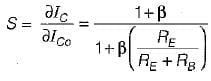Now,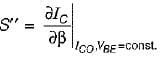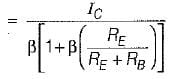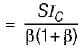QUESTION: 12

The forward current gain (α) of a bipolar transistor can be increased by
1. reducing the recombination lifetime in the emitter
2. increasing the emitter doping density.
3. increasing the base doping density.
4. reducing the base width.
Select the correct code from the given options,

Solution:

• When emitter is heavily doped, more majority carriers will reach into base due to which lC will increase and hence α increases.
• Due to early effect, base width is reduced as a result of which IC is slightly increased. Hence, α of the transistor is increased.
Hence, 2 and 4 are correct.

QUESTION: 13

What is the value of thermal resistance for the 2N338 transistor for which the manufacturer specifies PC,max = 125 mW at 25°C free-air temperature and maximum junction temperature of 150°C?

Solution:

Given,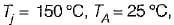PC,max = 125 mW
We know that,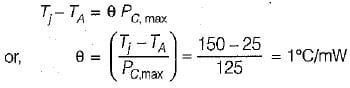QUESTION: 14

The stability factor (S1) for the circuit shown below is given by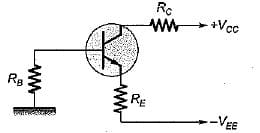Solution:

Applying KVL, we get: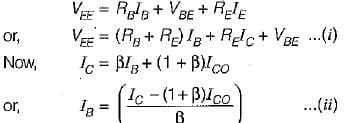Putting value of lB from equation (ii) into (i), we get: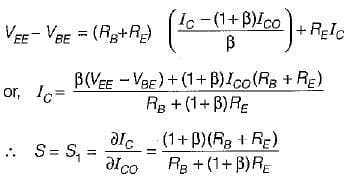QUESTION: 15

For the cascade amplifier shown below, the quiescent current through transistors is 1.mA and the collector voltage VC2 = 6 V and VC1 = 3 V. Assuming VBE = 0.7 V and very high Value of β, the values of R1 and R2 will be respectively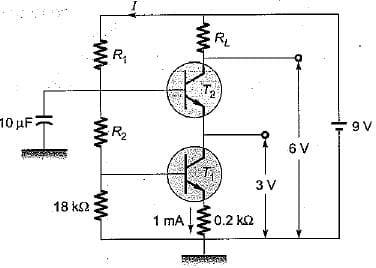Solution:

Since β is very large, we can neglect the base current of the transistors.
Now,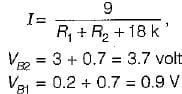Hence, current through 18 kΩ resistor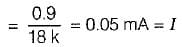∴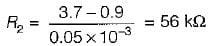and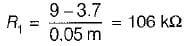Use Code STAYHOME200 and get INR 200 additional OFF Use Coupon Code